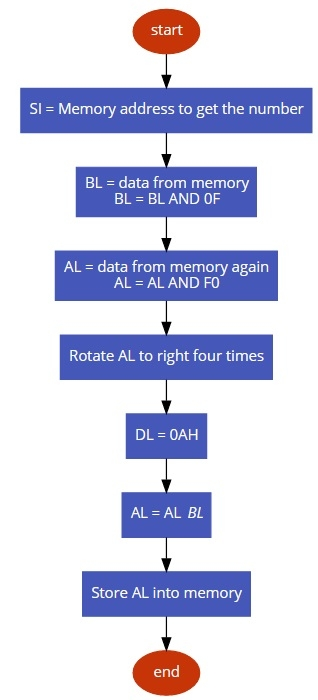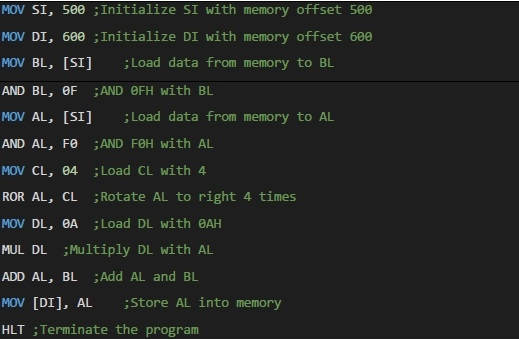# 8086 program to convert an 8 bit BCD number into hexadecimal number

In this program we will see how to find the equivalent hexadecimal number from a BCD number.

## Problem Statement

Write 8086 Assembly language program to find the equivalent hexadecimal number from a BCD number. The number is stored at memory offset 500 and store the result at memory offset 600.

## Discussion

To convert BCD to hexadecimal at first we have to cut the BCD digits. The most significant digit will be multiplied with 0AH (10D), and then least significant digit will be added with the result of multiplication. Thus the BCD will be converted to its equivalent hexadecimal form.

Data

500
59

## Flow Diagram## Program